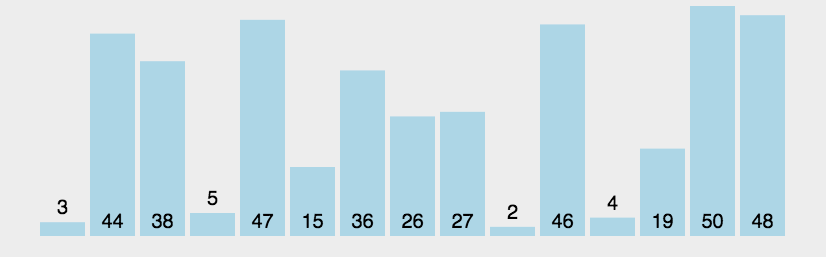# # 介绍

TIP

## # 时间复杂度

• 时间复杂度，又称时间复杂性，常用“大 O”符号表述，简称“大 O 表示法”
• 算法的时间复杂度是一个函数，它定性描述该算法的运行时间
• 定义为 T[n] = O(f(n)，称函数 T(n)以 f(n)为界或者称 T(n)受限于 f(n)
• 如果一个问题的规模是 n，解这一问题的某一算法所需要的时间为 T(n)，T(n)称为这一算法的“时间复杂度”
• 当输入量 n 逐渐加大时，时间复杂度的极限情形称为算法的“渐近时间复杂度”。

O(1)常数
O(log(n))对数
O(n)线性
O(nlog(n))线性和对数乘积
O(n²)平方
O(2n)指数• 规则一：用常量 1 取代运行时间中所有的加法常量
1. 如 7 + 8 = 15，用 1 表示运算结果 15，大 O 表示法表示为 O(1)
• 规则二：运算中只保留最高阶项
1. 如 n^{3} + 3n +1，大 O 表示法表示为：O(N3)
• 规则三：若最高阶项的常数不为 1，可将其省略
1. 如 4N2，大 O 表示法表示为：O(N2)

## # 算法效率